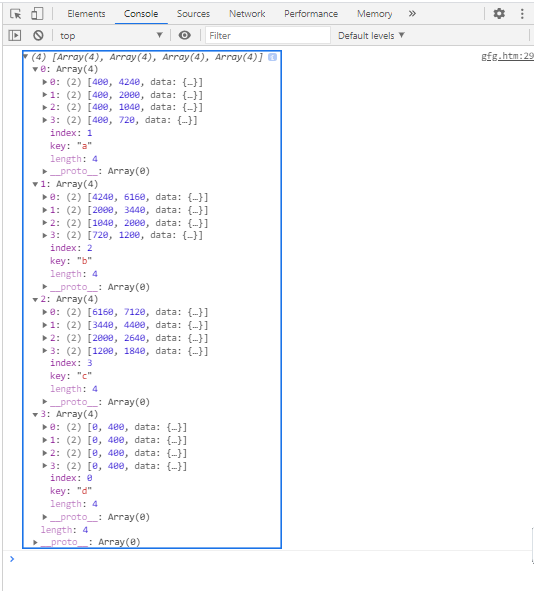GeeksforGeeks App
Open AppBrowser
Continue

# D3.js stackOrderInsideOut() Method

The D3.js stackOrderInsideOut() method returns a series order such that the larger series (according to the sum of values) are on the inside and the smaller series are on the outside.

Syntax:

`d3.stackOrderInsideOut(series);`

Parameters: This function accepts a single parameter as mentioned above and described below.

• series: This is the series based on the ordering of the keys to be ordered.

Return Value: This method returns no value.

Example: The following example demonstrates ordering the stack using d3.stackOrderInsideOut() function.

## HTML

 ```<``html``>``<``head``>``    ``<``meta` `charset``=``"utf-8"``>``    ``<``script` `src``=``        ``"https://d3js.org/d3.v5.min.js"``>``    `````` ` `<``body``>``    ``<``h1` `style``=``"text-align: center; color: green;"``>``        ``GeeksforGeeks``    ```` ` `    ``<``script``>``        ``var data = [``        ``{letter: {a: 3840, b: 1920, c: 960, d: 400}},``        ``{letter: {a: 1600, b: 1440, c: 960, d: 400}},``        ``{letter: {a: 640, b: 960, c: 640, d: 400}},``        ``{letter: {a: 320, b: 480, c: 640, d: 400}}``        ``];``        ``var stack = d3.stack()``            ``.keys(["a", "b", "c", "d"])``            ``.value((d, key) => d.letter[key])``            ``.order(d3.stackOrderInsideOut);`` ` `        ``var series = stack(data);``        ``console.log(series);``    `````` ` ``

Output:My Personal Notes arrow_drop_up
Related Tutorials